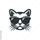cancel
Showing results for
Did you mean:Frequent Visitor

## Comparing Difference between two columns in two different tables

Hello, I have two tables that I am needing to compare and find the difference between.

Specifically, the tables have one column that should have matching values (ticket numbers), if the ticket numbers match then it should calculate the difference in the volumn column between the two matching ticket numbers. If the ticket number has no match I would also like to be able to note that.

I have tried a few different solutions on here in the last few days and can't seem to make it work.

Example data:

Table 1:

 Ticket Number Volume Taken Ticket Date 333 5 1/1/23 222 3 1/5/23 111 1 1/7/23

Table 2:

 Ticket Number Volume Ticket Date 333 4.5 1/1/23 222 1.0 1/5/23 100 1 1/7/23

Ideal Solution:

 Matching Ticket Number Table One Volume Table Two Volume Difference 333 5 4.5 -.5 222 3 1 -2.0 444 3 3 0

Ideally, I would like these two tables to compare and be able to make a graph showing that Ticket 333 had a volume difference of .5, ticket 222 had a volume difference of 2, and on a separate graph show that ticket number 111 had no matches from the other table.

Is something like this possible? If so could someone help me get started with this? Thank you!

1 ACCEPTED SOLUTIONSuper User

Please create a dimension table that contains all ticket numbers. This can be created in power query or can be a calculated table using the following dax

Tickets =
DISTINCT (
UNION (
ALLNOBLANKROW ( Table1[Ticket Num] ),
ALLNOBLANKROW ( Table2[Ticket Num] )
)
)
then you apply the following measure

Difference =
COALESCE (
SUMX (
VALUES ( Tickets[Ticket Num] ),
CALCULATE (
VAR Val1 =
SUM ( Table1[Value] )
VAR Val2 =
SUM ( Table1[Value] )
RETURN
IF ( Val1 <> BLANK () && Val2 <> BLANK (), Val2 - Val1 )
)
),
"No Match"
)

10 REPLIES 10Super User

Please create a dimension table that contains all ticket numbers. This can be created in power query or can be a calculated table using the following dax

Tickets =
DISTINCT (
UNION (
ALLNOBLANKROW ( Table1[Ticket Num] ),
ALLNOBLANKROW ( Table2[Ticket Num] )
)
)
then you apply the following measure

Difference =
COALESCE (
SUMX (
VALUES ( Tickets[Ticket Num] ),
CALCULATE (
VAR Val1 =
SUM ( Table1[Value] )
VAR Val2 =
SUM ( Table1[Value] )
RETURN
IF ( Val1 <> BLANK () && Val2 <> BLANK (), Val2 - Val1 )
)
),
"No Match"
)Frequent Visitor

Hey there!

I believe this almost has it. But at the moment it is showing the same difference for each ticket number. Let me know if anything stands out to you as something that needs changing. Thanks for your help!Super User

Have you created the relationship between the dimtable and the other two tables?Frequent Visitor

That's what I was missing, thanks so much!Helper I

First we need to create a relationship between the two tables, common Column is Tikcket number.

Second, we match the values or ticket numbers by creating calculated column so

m4 =
var _LookMtaches =
LOOKUPVALUE(Table1[Ticket Num],Table2[Ticket Num],Table1[Ticket Num])
Return
_LookMtaches
Third we Calculate the difference =
M3 =
CALCULATE(SUM(Table1[Volume])-SUM(Table2[Volume]))
M4, Volume from table 1, volume from table 2, M3.
Please note: 1- you might have empty columns so Drag M4 to filter panel and choose is not blank.
2- When drag M4 to the summary table choose "don't summarize"
I hope this helps and please check the answer if it is rightFrequent Visitor

Hey Galaamri, thank you for being willing to help. I do believe this would be the solution if I could get the relationship to work.

When creating m4, I get the error "The column 'table 2 [ticket number]' either doesn't exist or doesn't have a relationship to any table available in the current context".

Extra info in case any of these help with solving the errors:
-I am attempting to create the calculated column column inside of table 1
-The ticket numbers from table 1 and table 2 will not match, a lot will but a lot will not. Some also include characters instead of just numbers. Both ticket columns are data type "text".
-When creating a relationship between the two before attempting to create m4, it makes a "many to many" relationship".Helper I

Then there is other way to make this relationship works by creating a reference table (to just have the unique ticket numbers). to do this:

Go to Power Query (Transform data), home, manage, then choose referece. you are creating a reference table1 based on the ticket number. then remove duplicate. then do the same for table2.

Connect the reference table1 and table1 (This should have one to many relationship), and the mesaure would work.

RegardsFrequent Visitor

Unfortunately it is still many to many relationship (I assume due to Table 1 will have ticket numbers table 2 doesn't have, and vice versa). But I am not entirely sure of the cause. But I do really appreciate the help.Resolver I

Hi @powerbiqu ,

This is how you get the difference...

``Difference = SUM('Table 1'[Volume]) - SUM('Table 2'[Volume])``

Make sure Ticket Number is type Text or it won't work in your graph properly.

Many Thanks,

Taylor😎Frequent Visitor

Hey,

Thank you for helping but I am needing to first match the values from one column before getting the difference between the correlating second columns.

So if Ticket Number has a matching value in the second table, I want to compare the volumes in the two tables that correspond to that specific ticket number.